# Electronics and Communication Engineering - Exam Questions Papers

46.

A surface charge density of 8 nc/m2 is present on a plane x = z. A line charge density of 30 nC/m is present on line x = 1, y = 2 Find VAB for points A(3, 4, 0) and B(4, 0, 1)

 A. 583.38 V B. 58.338 V C. 583.38 mV D. 58.338 mV

Explanation:

Potential due to surface charge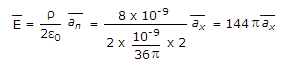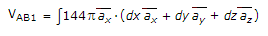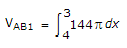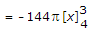= 144pv = 452.16v

Potential due to line charge at x = 1 and y = 2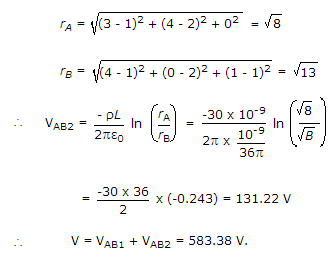47.

Which of the following is equivalent to x?

 A. x EX-OR 0 B. x EX-NOR 0 C. 1 NOR x D. x AND x

Explanation:

Truth table of X-NOR gate :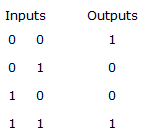i.e. when an input to the X-NOR gate is '0' then the other input to the gate is complemented at the output.

48.

The electric field vector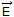of a wave in free space (εo, μo) is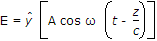Its magnetic field vector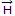will be given by

 A.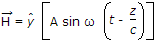B.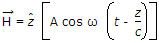C.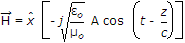D.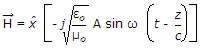Explanation: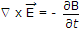Now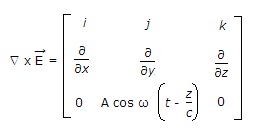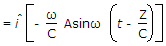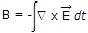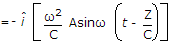(use B = μ0 H) .

49.

A uniform plane wave is described by the equation H =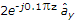A/m. If the velocity of the wave is 2 x 108 m/s and εr = 1.8, then. The frequency of the wave is

 A. 1.5 x 106 Hz B. 1.5 x 108 Hz C. 106 Hz D. 107 Hz

Explanation: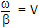r = 0.1p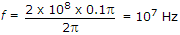.

50.

Consider two random processes x(t) and y(t) have zero mean, and they are individually stationary. The random process is z(t) = x(t) + y(t). Now when stationary processes are uncorrelated then power spectral density of z(t) is given by

 A. Sx(f) + Sy(f) + 2Sxy(f) B. Sx(f) + Sy(f) + 2Sxy(f) + 2Syx(f) C. Sx(f) + Sy(f) D. Sx(f) + Sy(f) - 2Sxy(f) - 2Syx(f)

Explanation:

The autocorrelation function of z(t) is given by

Rz(t, u) = E[Z(t)Z(u)]

= E[(x(t) + y(t)) (x(u) + y(u))]

= E[x(t) x (u)] + E[x(t) y(u)] + E[y(t) x(u)] + E[y(t) y(u)]

= Rx(E, u) + Rxy(t, u) + Ryx(t, u) + Ry(t, u)

Defining t = t - u, we may therefore write Rz(t) = Rx(t) + Rxy(t) + Ryx(t) + Ry(t).

When the random process x(t) and y(t) are also jointly stationary.

Accordingly, taking the fourier transform of both sides of equation we get

Sz(f = Sx(f) + Sxy(f) + Syx(f) + Sy(f)

We thus see that the cross spectral densities Sxy(f) and Syx(f) represent the spectral components that must be added to the individual power spectral densities of a pair of correlated random processes in order to obtain the power spectral density of their sum.

When the stationary process x(t) and y(t) are uncorrelated the cross-sectional densities Sxy(f) and Syz(f) are zero

Sz(f) = Sx(f) + Sy(f).

#### Current Affairs 2022

Interview Questions and Answers